# CALCULATOR TRAINING FOR THE EOC CASIO fx260 Solar

• Slides: 26
Download presentationCALCULATOR TRAINING FOR THE EOC CASIO fx-260 SolarTo the basics…. . Turn your calculator ON1) ADDITION 3) MULTIPLICATION Type 23 + 12 = Type 33 X 20 = 35, but you knew that. Press AC a couple of times after each operation. 2) SUBTRACTION Type 89 – 35 = 54, but you knew that too 660, but you knew that (right? ) 4) DIVISION Type 45 ÷ 9 = 5, of course!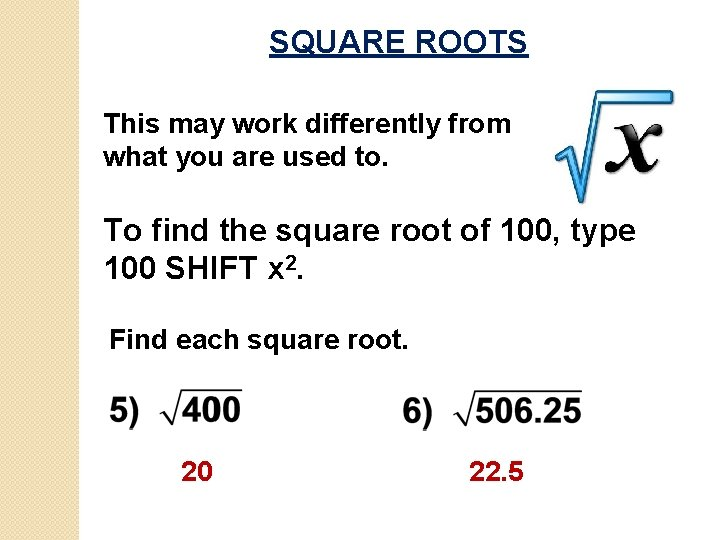SQUARE ROOTS This may work differently from what you are used to. To find the square root of 100, type 100 SHIFT x 2. Find each square root. 20 22. 5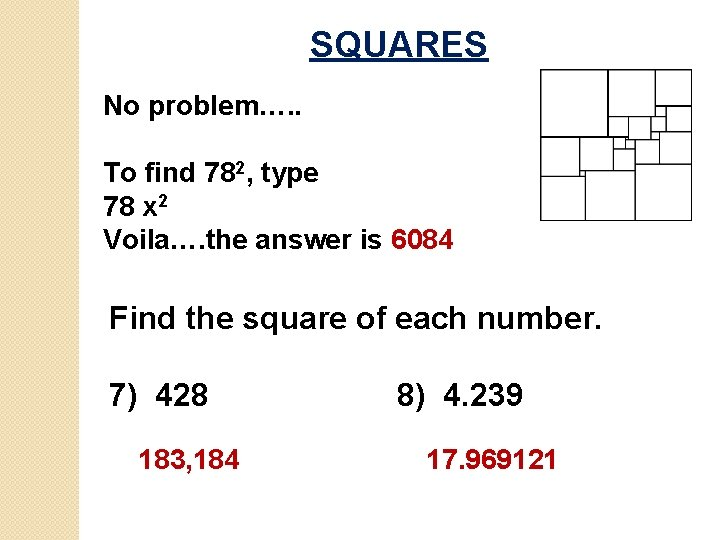SQUARES No problem…. . To find 782, type 78 x 2 Voila…. the answer is 6084 Find the square of each number. 7) 428 183, 184 8) 4. 239 17. 969121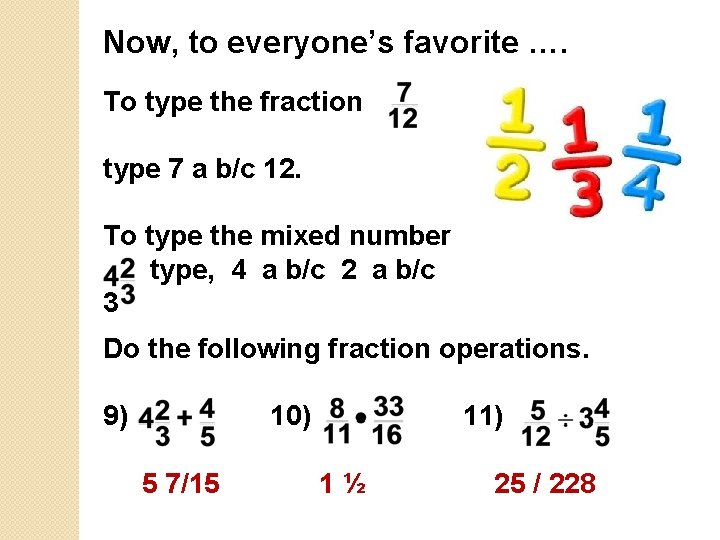Now, to everyone’s favorite …. To type the fraction type 7 a b/c 12. To type the mixed number type, 4 a b/c 2 a b/c 3 Do the following fraction operations. 9) 10) 5 7/15 11) 1½ 25 / 228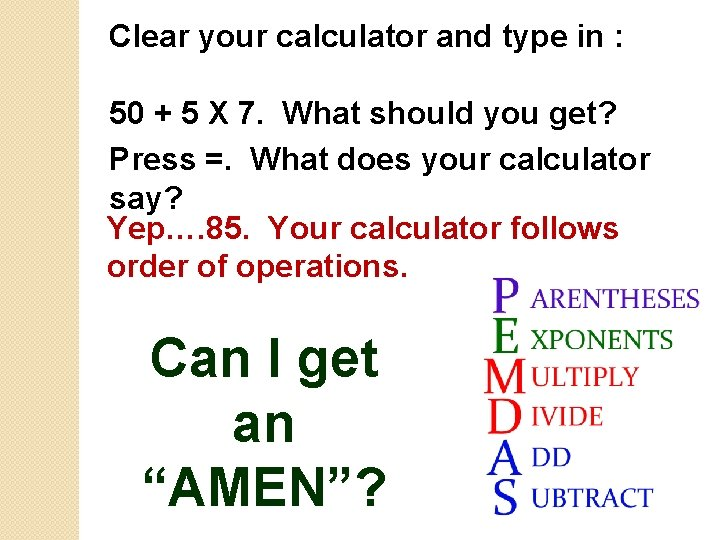Clear your calculator and type in : 50 + 5 X 7. What should you get? Press =. What does your calculator say? Yep…. 85. Your calculator follows order of operations. Can I get an “AMEN”?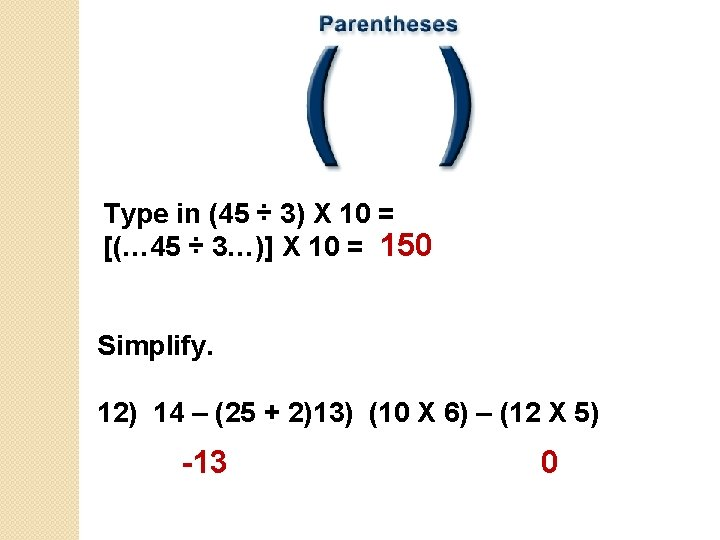Type in (45 ÷ 3) X 10 = [(… 45 ÷ 3…)] X 10 = 150 Simplify. 12) 14 – (25 + 2)13) (10 X 6) – (12 X 5) -13 0Let’s move up a dimension and work with To find 203, type in 20 SHIFT. You should get 8000. Find 14) 113 1331 15) (-16)3 -4096 NOTE : type [(… 16 +/…. )]SHIFT The +/- button changes the sign of the number.LARGER EXPONENTS To find 134, type in 13 xy 4 =. You should get 28, 561. Find 16) 95 17) (-3)8 59, 049 6561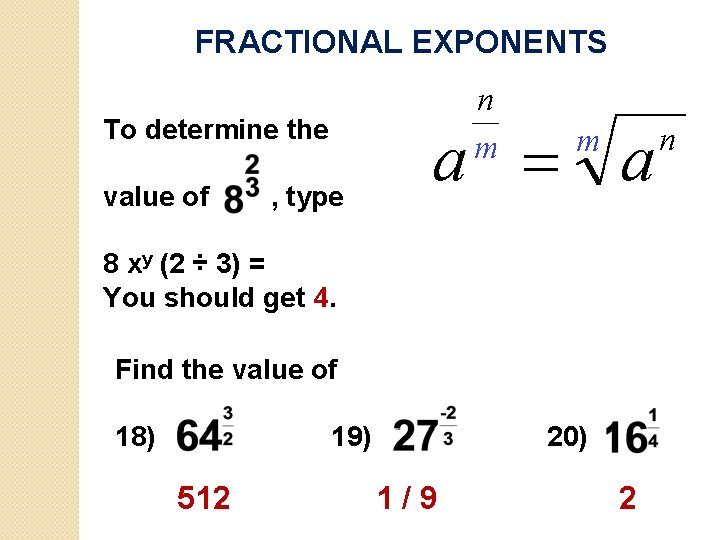FRACTIONAL EXPONENTS To determine the value of , type 8 xy (2 ÷ 3) = You should get 4. Find the value of 18) 19) 512 20) 1/9 2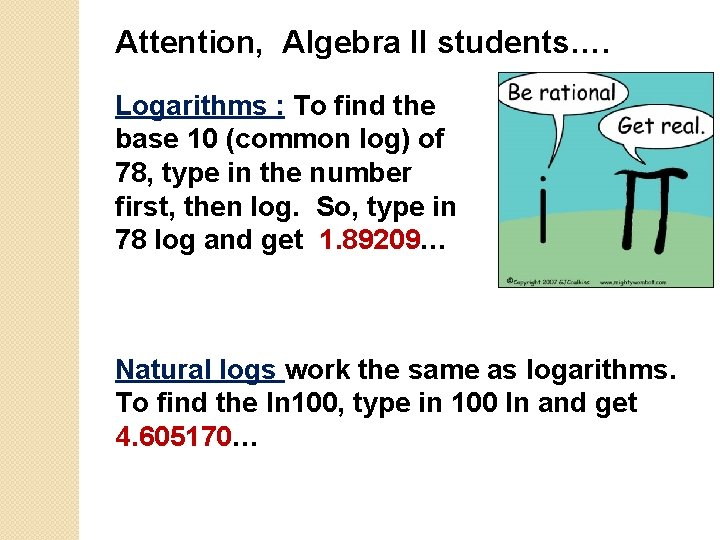Attention, Algebra II students…. Logarithms : To find the base 10 (common log) of 78, type in the number first, then log. So, type in 78 log and get 1. 89209… Natural logs work the same as logarithms. To find the ln 100, type in 100 ln and get 4. 605170…To find a power of 10, that is find 105. Type in the exponent first, then SHIFT 10 x. Type in 5 SHIFT 10 x and get 100, 000. To find a power of e, again, type in the exponent first, then SHIFT ex. So, e 10 SHIFT ex equals 22, 026. 46579…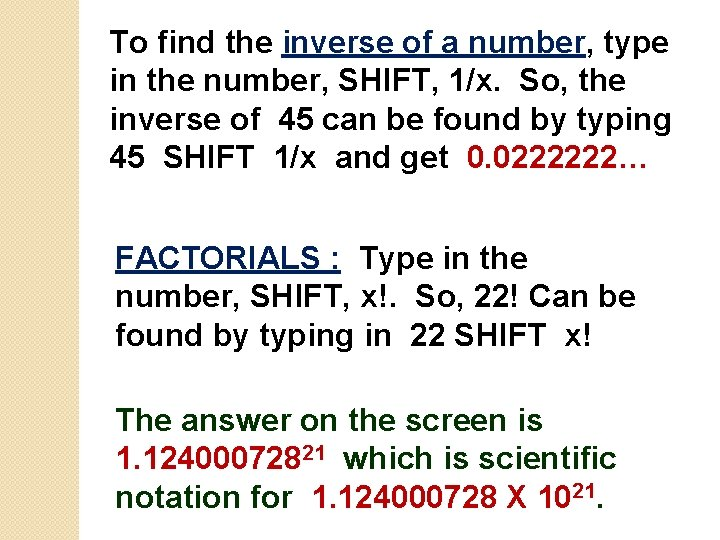To find the inverse of a number, type in the number, SHIFT, 1/x. So, the inverse of 45 can be found by typing 45 SHIFT 1/x and get 0. 0222222… FACTORIALS : Type in the number, SHIFT, x!. So, 22! Can be found by typing in 22 SHIFT x! The answer on the screen is 1. 12400072821 which is scientific notation for 1. 124000728 X 1021.COMBINATIONS : To find 20 C 14, type in 20 SHIFT n. Cr 14 = to get 38, 760 PERMUTATIONS : Work the same as combinations, though you use n. Pr for permutations.TRIGONOMETRY … YIPPEE!!! Matching 1) Sine 2) Cosine 3) Tangent Correct Answers: 1) C 2) A 3) B a) adjacent / hypotenuse b) opposite / adjacent c) opposite / hypotenuse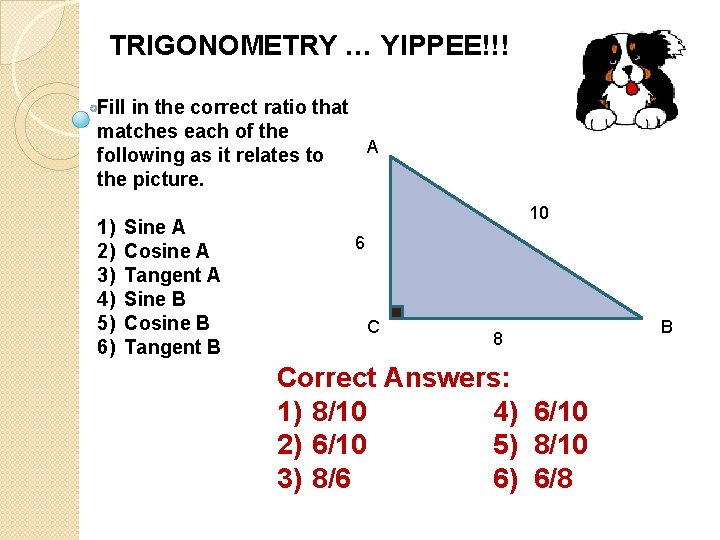TRIGONOMETRY … YIPPEE!!! Fill in the correct ratio that matches each of the A following as it relates to the picture. 1) 2) 3) 4) 5) 6) Sine A Cosine A Tangent A Sine B Cosine B Tangent B 10 6 C 8 Correct Answers: 1) 8/10 4) 6/10 2) 6/10 5) 8/10 3) 8/6 6) 6/8 B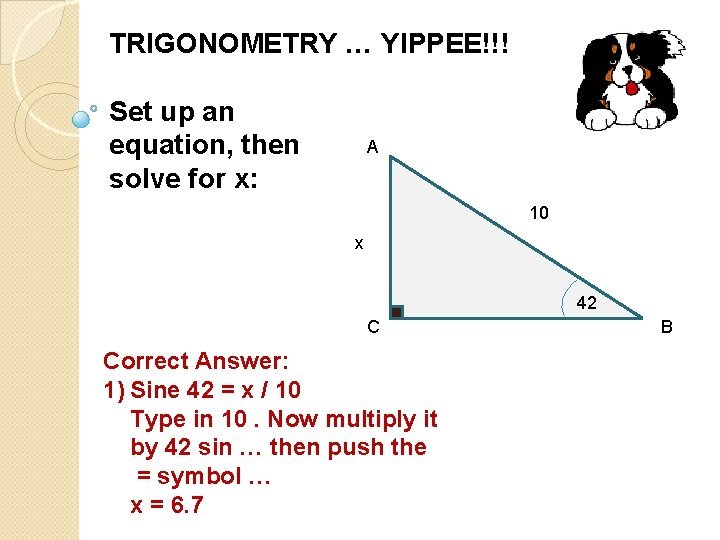TRIGONOMETRY … YIPPEE!!! Set up an equation, then solve for x: A 10 x 42 C Correct Answer: 1) Sine 42 = x / 10 Type in 10. Now multiply it by 42 sin … then push the = symbol … x = 6. 7 B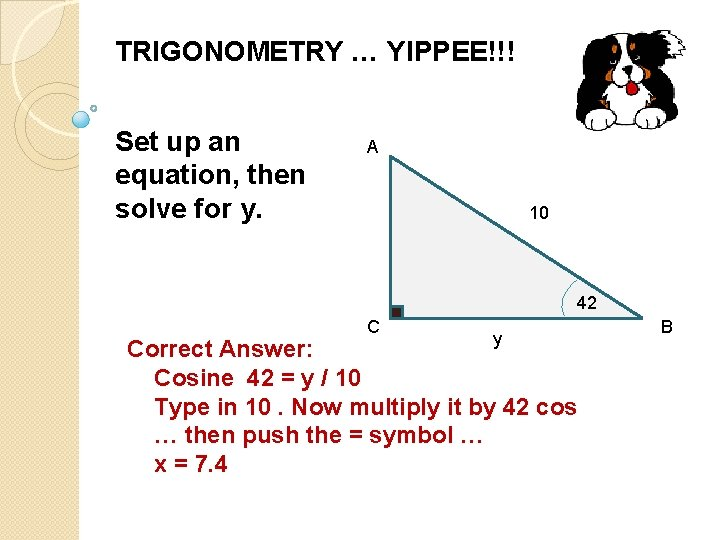TRIGONOMETRY … YIPPEE!!! Set up an equation, then solve for y. A 10 42 C y Correct Answer: Cosine 42 = y / 10 Type in 10. Now multiply it by 42 cos … then push the = symbol … x = 7. 4 B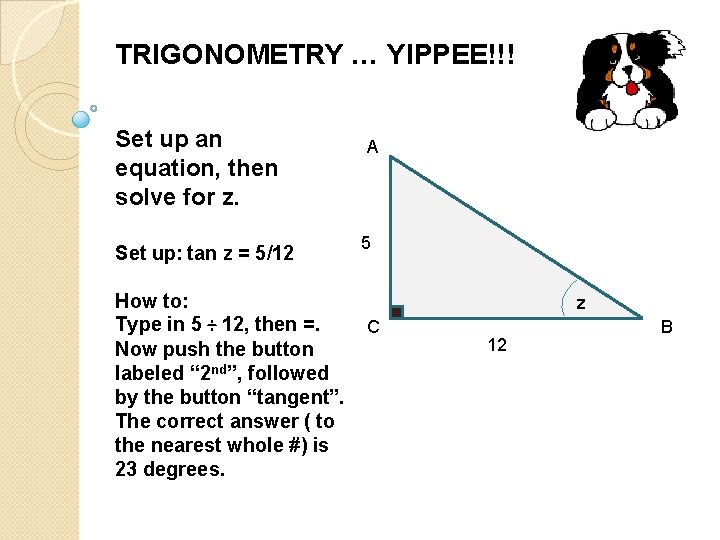TRIGONOMETRY … YIPPEE!!! Set up an equation, then solve for z. Set up: tan z = 5/12 How to: Type in 5 ÷ 12, then =. Now push the button labeled “ 2 nd”, followed by the button “tangent”. The correct answer ( to the nearest whole #) is 23 degrees. A 5 z C 12 B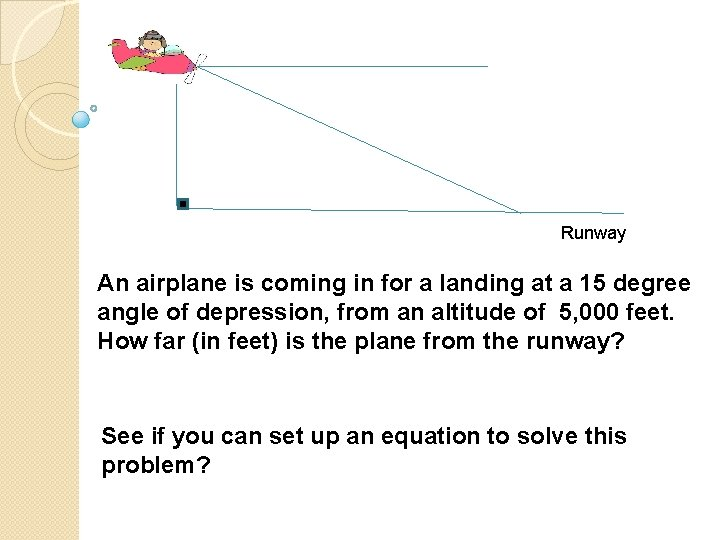Runway An airplane is coming in for a landing at a 15 degree angle of depression, from an altitude of 5, 000 feet. How far (in feet) is the plane from the runway? See if you can set up an equation to solve this problem?15 5000 x 15 Runway Sine = opposite / hypotenuse Sine 15 = 5000 / x Now see if you can solve the equation & determine how far the plane is from the runway (to the nearest foot).15 5000 x 15 Runway sin 15 = 5000 / x sin 15 (x) = 5000 x = 5000 / sin 15 On your calculator, you should have typed in the number 5000 first. Now push the ÷ symbol and the number 15, followed the button Sine. Now push the = symbol to get the answer of 19, 319 feet (approx 3. 7 miles)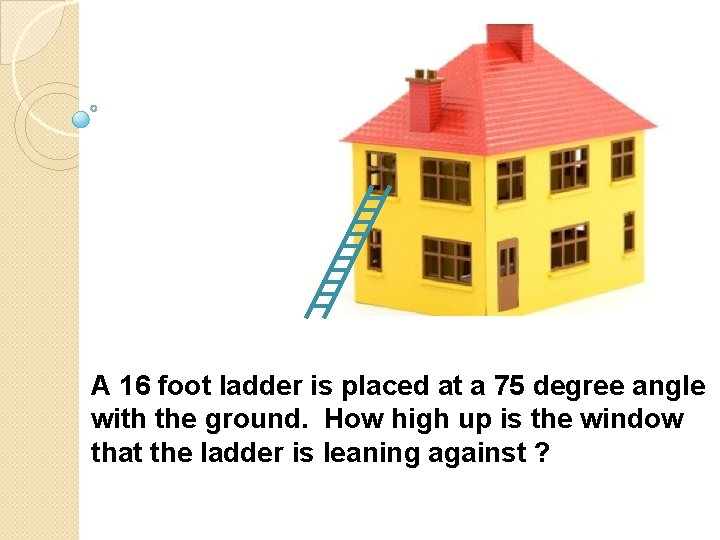A 16 foot ladder is placed at a 75 degree angle with the ground. How high up is the window that the ladder is leaning against ?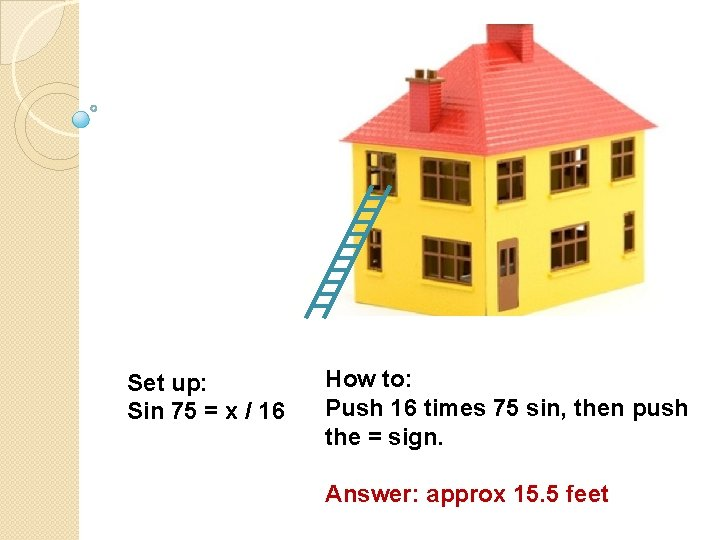Set up: Sin 75 = x / 16 How to: Push 16 times 75 sin, then push the = sign. Answer: approx 15. 5 feetEQUATION EDITOR TUTORIAL http: //demo. tds. airast. org/eqtu torial/? c=florida_pt&language =true